# Comprehensive Classification Series – Kaggle’s Titanic Problem Part 2 :Understanding The Data and Exploratory Data Analysis with VisualizationsShare this article!

# Understanding The Data and Exploratory Data Analysis with Visualizations

This is the second part of the Comprehensive Classification Series.
We strongly recommend you to go through the previous parts before starting with this one.
The series is as follows:

Part 1 – Introduction to Kaggle

Part 2 – Understanding The Data and Exploratory Data Analysis(this article

The Titanic challenge on Kaggle is a competition in which the goal is to predict the survival or the death of a given passenger based on a set of variables describing him such as his age, his sex, or his passenger class on the boat.
Through this part of the tutorial our aim is to understand the data set and the problem.

I hope you are familiar with the term EDA – Exploratory Data Analysis you can find more details here in our another post EDA.

So lets begin and import the libraries we will be using.

A very easy way to install these packages is to download and install the Conda distribution that encapsulates them all. This distribution is available on all platforms (Windows, Linux and Mac OSX).

In :
```import pandas as pd
import numpy as np
import seaborn as sns
import matplotlib.pyplot as plt
import matplotlib
matplotlib.style.use('ggplot')

%matplotlib inline
```

Two data sets are available: a training set and a test set. We’ll be using the training set to build our predictive model and the testing set to score it and generate an output file to submit on the Kaggle evaluation system.
We’ll see how this procedure is done at the end of this series.
Now let’s start by loading the training set.

So first lets load the data , pandas provides us many ways in which we can load our files and it extends to many file formats

In :
```data= pd.read_csv("train.csv")
```

Yes , a single line of code to read the data in. Now lets use the head method to peak into our data

In :
```data.head()
```
Out:
PassengerId Survived Pclass Name Sex Age SibSp Parch Ticket Fare Cabin Embarked
0 1 0 3 Braund, Mr. Owen Harris male 22.0 1 0 A/5 21171 7.2500 NaN S
1 2 1 1 Cumings, Mrs. John Bradley (Florence Briggs Th… female 38.0 1 0 PC 17599 71.2833 C85 C
2 3 1 3 Heikkinen, Miss. Laina female 26.0 0 0 STON/O2. 3101282 7.9250 NaN S
3 4 1 1 Futrelle, Mrs. Jacques Heath (Lily May Peel) female 35.0 1 0 113803 53.1000 C123 S
4 5 0 3 Allen, Mr. William Henry male 35.0 0 0 373450 8.0500 NaN S

This helps to get into the top 5 rows of our data set, you can similarly use tail to check out the bottom five.

Data Dictionary

Variable Definition Key
survival Survival 0 = No, 1 = Yes
pclass Ticket class 1 = 1st, 2 = 2nd, 3 = 3rd
sex Sex
Age Age in years
sibsp # of siblings / spouses aboard the Titanic
parch # of parents / children aboard the Titanic
ticket Ticket number
fare Passenger fare
cabin Cabin number
embarked Port of Embarkation C = Cherbourg, Q = Queenstown, S = Southampton
Variable Notes

pclass: A proxy for socio-economic status (SES)
1st = Upper
2nd = Middle
3rd = Lower

age: Age is fractional if less than 1. If the age is estimated, is it in the form of xx.5

sibsp: The dataset defines family relations in this way…
Sibling = brother, sister, stepbrother, stepsister
Spouse = husband, wife (mistresses and fiancés were ignored)

parch: The data set defines family relations in this way…
Parent = mother, father
Child = daughter, son, stepdaughter, stepson
Some children traveled only with a nanny, therefore parch=0 for them.

Lets check the shape of the data, that is the number of rows and the number of coulmns, this gives us the rough idea about our data

In :
```data.shape
```
Out:
`(891, 12)`

Pandas allows you to statistically describe numerical features using the describe method.

In :
```data.describe()
```
Out:
PassengerId Survived Pclass Age SibSp Parch Fare
count 891.000000 891.000000 891.000000 714.000000 891.000000 891.000000 891.000000
mean 446.000000 0.383838 2.308642 29.699118 0.523008 0.381594 32.204208
std 257.353842 0.486592 0.836071 14.526497 1.102743 0.806057 49.693429
min 1.000000 0.000000 1.000000 0.420000 0.000000 0.000000 0.000000
25% 223.500000 0.000000 2.000000 20.125000 0.000000 0.000000 7.910400
50% 446.000000 0.000000 3.000000 28.000000 0.000000 0.000000 14.454200
75% 668.500000 1.000000 3.000000 38.000000 1.000000 0.000000 31.000000
max 891.000000 1.000000 3.000000 80.000000 8.000000 6.000000 512.329200

The count variable shows that 177 values are missing in the Age column.
One solution is to replace the null values with the median age which is more robust to outliers than the mean

In :
```data['Age'].fillna(data['Age'].median() , inplace= True )
```

Lets check that out again.

In :
```data.describe()
```
Out:
PassengerId Survived Pclass Age SibSp Parch Fare
count 891.000000 891.000000 891.000000 891.000000 891.000000 891.000000 891.000000
mean 446.000000 0.383838 2.308642 29.361582 0.523008 0.381594 32.204208
std 257.353842 0.486592 0.836071 13.019697 1.102743 0.806057 49.693429
min 1.000000 0.000000 1.000000 0.420000 0.000000 0.000000 0.000000
25% 223.500000 0.000000 2.000000 22.000000 0.000000 0.000000 7.910400
50% 446.000000 0.000000 3.000000 28.000000 0.000000 0.000000 14.454200
75% 668.500000 1.000000 3.000000 35.000000 1.000000 0.000000 31.000000
max 891.000000 1.000000 3.000000 80.000000 8.000000 6.000000 512.329200

Perfect. Now we have a fair idea of what the data set looks like and what each column represents, lets dive in a bit with visualizations and lets analyze this data.

Lets take up gender as our first criteria-

In :
```sns.countplot(x='Survived',data=data , hue ='Sex' )
```
Out:
`<matplotlib.axes._subplots.AxesSubplot at 0x155587720f0>`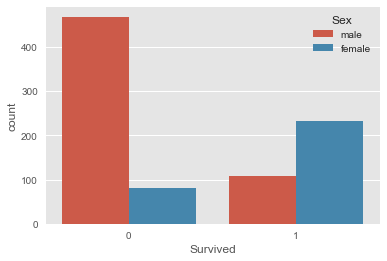The Sex variable seems to be a decisive feature. Women are more likely to survive.
After this what comes to our mind is the age of the survives. So lets use age to correlate

In :
```fig =plt.figure(figsize=(12,9))
plt.hist( [data[data['Survived']==1]['Age'] , data[data['Survived'] ==0]['Age']] ,stacked=True , color = ['g','r'],
bins = 30,label = ['Survived','Dead'])
plt.xlabel('Age')
plt.ylabel('Number of passengers')
plt.legend()
```
Out:
`<matplotlib.legend.Legend at 0x1555893e278>`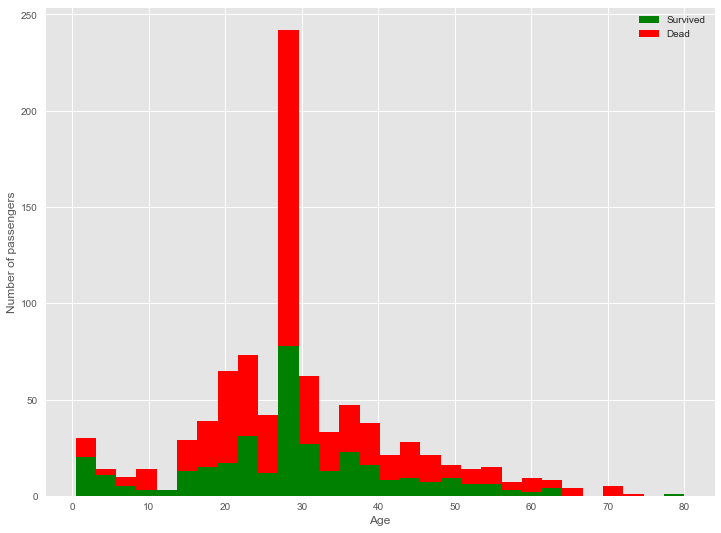If you go through this plot you will realize the survival rate is max in the age group 0-12 which clearly indicates children and female passengers ere the first preference.

Another feature that we might consider here is FARE, is the amount of money paid for the ticket correlated to the survival of passengers , Lets check this hypothesis with another intuitive graph.

In :
```figure = plt.figure(figsize=(15,8))
plt.hist([data[data['Survived']==1]['Fare'],data[data['Survived']==0]['Fare']], stacked=True, color = ['g','r'],
bins = 50,label = ['Survived','Dead'])
plt.xlabel('Fare')
plt.ylabel('Number of passengers')
plt.legend()
```
Out:
`<matplotlib.legend.Legend at 0x1555893e5c0>`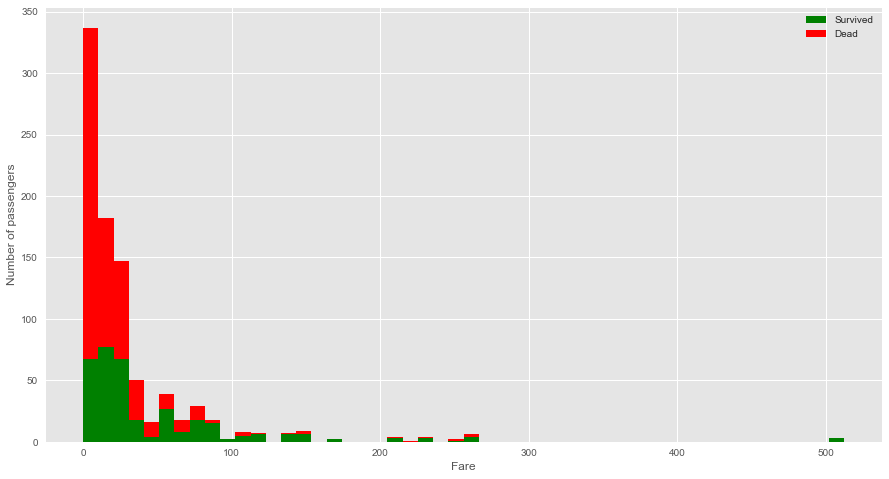Passengers with cheaper ticket fares are more likely to die. Put differently, passengers with more expensive tickets, and therefore a more important social status, seem to be rescued first.
Let’s now combine the age, the fare and the survival on a single chart.

In :
```plt.figure(figsize = (15,8))
ax = plt.subplot()
ax.scatter(data[data['Survived']==1]['Age'] , data[data['Survived']==1]['Fare'] , c='green' , s=40)
ax.scatter(data[data['Survived']==0]['Age'] , data[data['Survived']==0]['Fare'] , c='red' , s=40)
ax.set_xlabel('Age')
ax.set_ylabel('Fare')
```
Out:
`<matplotlib.text.Text at 0x15558d7b6a0>`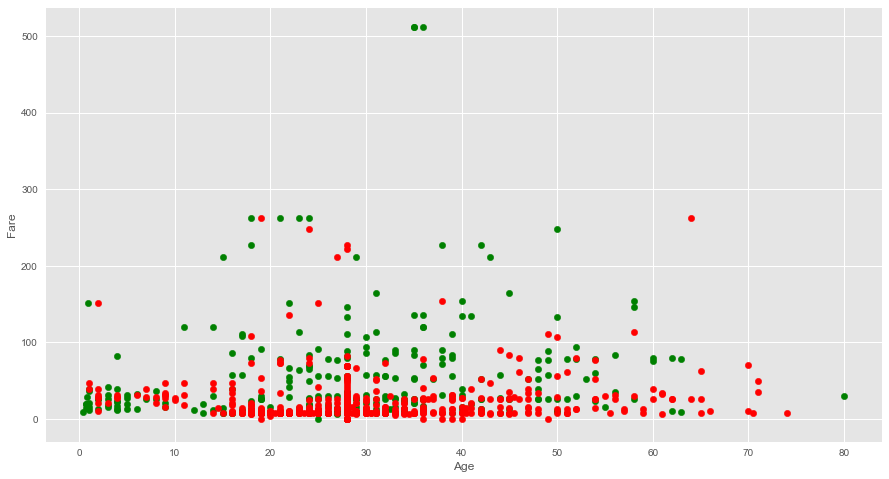The red cluster obtained is of the people who died, you can spot the green cluster near the origin , low fare and children, the green spots are more vibrant as the fare increases. Thus fare is an interesting feature to deal with.

Moving on another column that is class is also seems interesting too .

In :
```ax = plt.subplot()
ax.set_ylabel('Average fare')
data.groupby('Pclass').mean()['Fare'].plot(kind='bar',figsize=(15,8), ax = ax)
```
Out:
`<matplotlib.axes._subplots.AxesSubplot at 0x15558df9438>`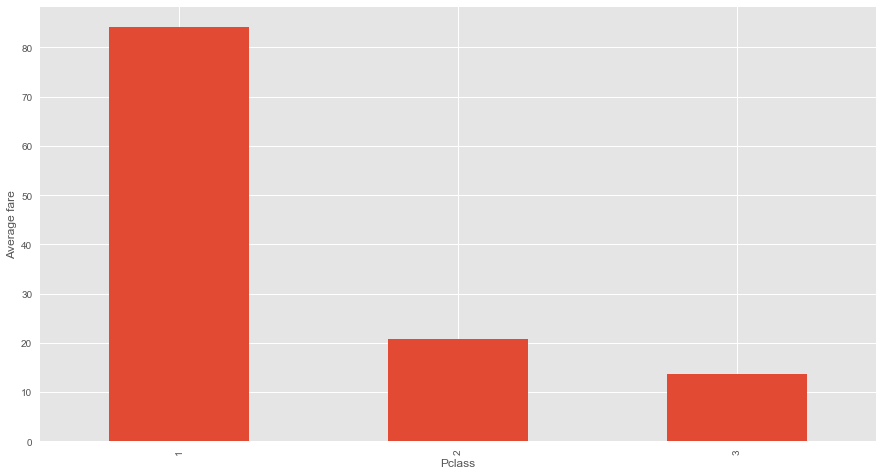Lets check that does the site of embarkation has any effect on survival, try and predict this before checking out the graph

In :
```sns.countplot('Survived' , data =data , hue ='Embarked')
```
Out:
`<matplotlib.axes._subplots.AxesSubplot at 0x1555923ab70>`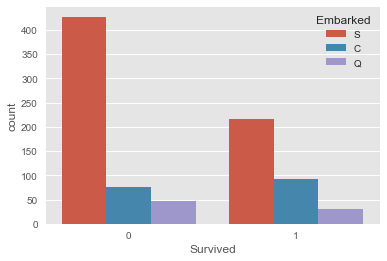There isn’t any specific correlation that we should look for.

To compare the numerical features of the data we use seaborn’s pair plot and see if we can find something interesting.

In :
```sns.pairplot(data)
```
Out:
`<seaborn.axisgrid.PairGrid at 0x155591e4e10>`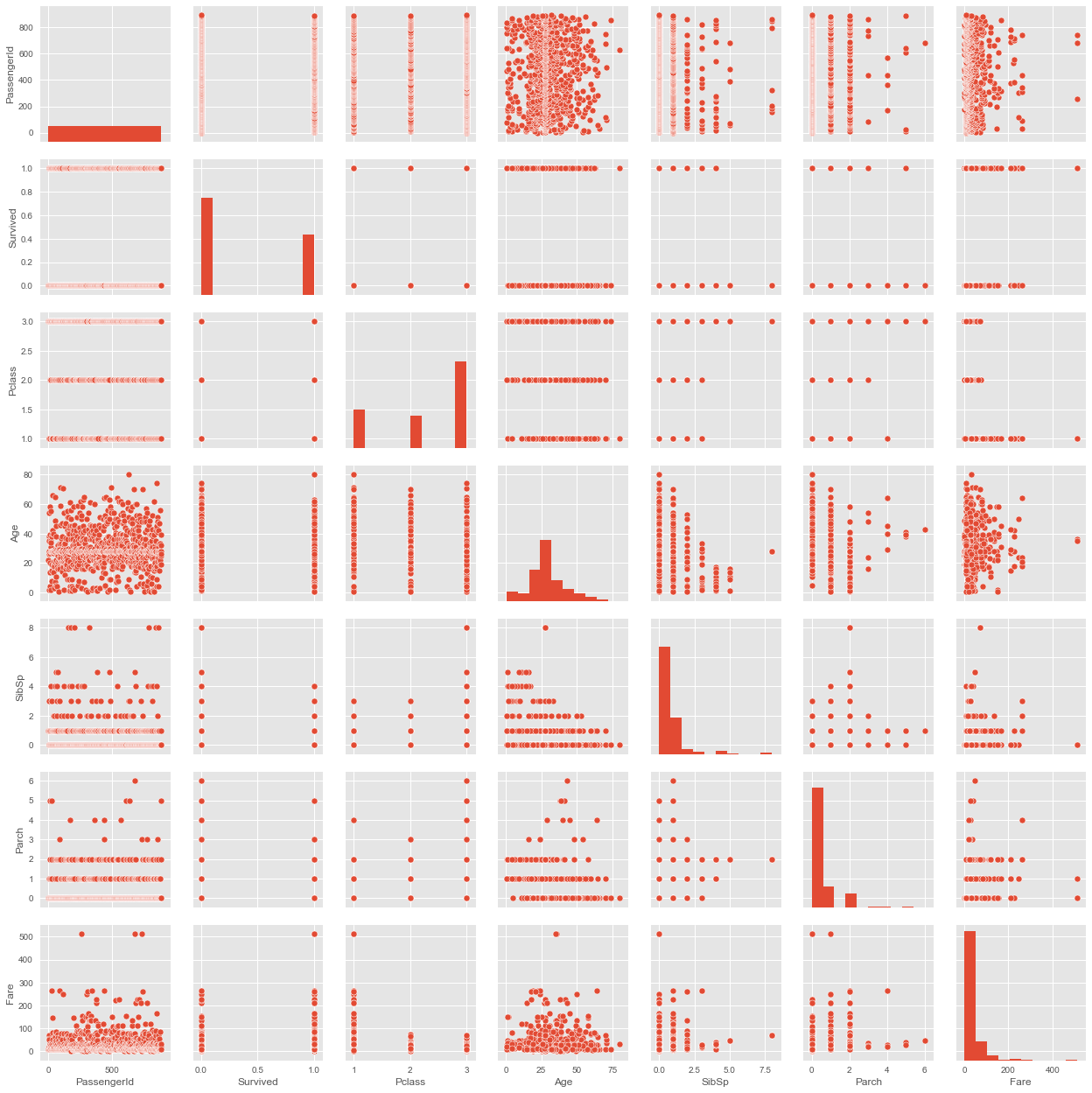Now your task is to get more visualization out of the data set and find out if another aspect is correlated too.

# Until Next Time

Our next task is to add features to our data to what we call as Feature Engineering. Then we will move on to build our model.

We hope you like this part of the series. Feel free to comment out your doubts.

Stay Tuned !!

Share this article!